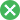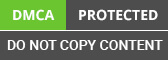﻿ Linear Algebra For Improving Machine Learning Skills

Looking for an Expert Development Team? Take two weeks Trial! Try Now

•Fill the details and We’ll get back to you soon# Understanding Machine Learning Algorithms using Linear Algebra## Why do we use math in Machine Learning?

Creating an algorithm that can learn from data to make a prediction is what Machine Learning is all about. Machine Learning is built on mathematical prerequisites, and sometimes it feels like learning them might be a bit overwhelming. If you want to know how machine learning works, then learning the math behind it will let you choose the best model that's your problem statement.

Machine Learning is Powered by Statistics, Calculus, Probability, and Linear Algebra. The core of everything is statistics. Optimize your model using calculus. Linear Algebra makes running of these algorithms feasible on Massive Data Sets, and Probability helps predict the likelihood of an event occurring.

The main object of linear algebra is matrices and vectors. Matrix is a rectangular collection of scalar values it has M rows and n columns, and we often denote the element at position IJ by matrix aij or sometimes by that small scaler aij

Linear Algebra is related to linear equations in mathematics

a1x1+…..+anxn=b

Vector Notation aTx=b

This is called a linear transformation of x

Linear Algebra is which defines objects and is a fundamental part of geometry

## Why do we need Linear Algebra?

Linear algebra is based on continuous mathematics, which is used through engineering. The Input Vectors are converted into a series of linear transformations.

### Scalar:

Scalars are a single number of objects. They are mainly real-valued or integers which are represented in lower-case italic x

### Vector:

Vectors are an array of numbers which are arranged in order. They are identified in by an index are represented in lower-case bold x Vectors are points in space where each element is plotted along an axis When one talks about machine learning Data Representation becomes an essential aspect of data analytics solutions, and data is represented usually in a matrix form. Having a good understanding of some of these concepts before you step in and understand more complicated or more sophisticated machine learning algorithms.

## What is a Matrix?

A matrix is a form of organizing data into rows and columns. There are many ways in which you can organize a data matrix to provide you a convenient way of organizing the data. So, if you are an engineer and looking at data for multiple variables, at multiple times, the matrix is very much helpful.

How do you put this data together in a format that can be used later, is what a matrix is helpful for? Matrices can be used to represent samples with multiple attributes in a compact form. Matric can also be used to represent linear equations compactly and straightforwardly. Linear algebra provides tools to understand and manipulate matrices to derive useful knowledge from data.

Usually, matrices are used to store and represent the data on machines. Matric is a very natural approach to organizing data. In general, rows represent the samples. Columns represent the values of the variables.

### Matrices

If Two indices identify each element of the matrices, then it is a 2-D Array of Numbers. It is Denoted by A

### Tensor

The RGB Color Image, which has three axes is an example of a Tensor. Tensor is an array of numbers which are arranged on the grid. This grid is regular and has a variable number of axes. Tensor is Denoted by A

If the Matrices have the same shape, then we can add the corresponding elements.

C = A+B

Ci,j = Ai,j +Bi,j

The Matrix can be added to the scalar or even multiplied

D= aB+c

Di,j = aBi,j +c

Matrix Multiplication

Matrix Transformation

Machine Learning- Flow of Tensors

Linear Equations

Matrix Formulation: Ax=b

Solution: x=A-1b

## Angle between Vectors

L2 norms are represented by the dot product, which is written in terms of angle θ between the two vectors.

### Diagonal Matrix:

The Diagonal Matrix has a Lot of Zeros, and the non-zero entries are only in the diagonals.

### Symmetric Matrix

A Symmetric Matrix is of the Form A=AT

Covariance is Symmetric

### Unit Vector

A Unit Vector is of the Form

## Future Analysis

We can represent the data into a matrix format, what are the questions we need to ask?

• Are all the attributes in the data matrix relevant?
• Is there any method which can identify if some attributes are related to the other attributes?
• If yes, how do we identify the linear relationship?
• Can we use this to reduce the size of the data matrix?

How does one identify the independent attributes?

• Domain Knowledge D is the function of P and T
• Implying that at least one attribute is dependent on the others
• Variable can be calculated as a linear combination of other variables

## The rank of a Matrix

Let us assume that we have many more samples than attributes for now. Is there any approach which can be used to identify the number of linear relationships between the attributes purely using data? The rank of a matrix refers to the number of linearly independent rows or columns of the matrix. The rank of the matrix is found by using the rank command: rank(A)

## Null Space: The Idea

Notice that if A β=0, every row of A when multiplied by β goes to zero. This implies that variable values in each sample behave the same. Every null space corresponds to one linear relationship.

Null Space: The Idea

The notion of rank gives you the notion of the number of independent variables or samples. We have touched upon some of the critical features of Machine learning development solutions and a real-world example which would have sparked the importance and power of learning machine learning. Start improving your machine learning skills by understanding linear algebra.